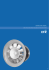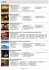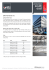# kucukarslan et al.

## Transcription

kucukarslan et al.
```International Journal of Applications of Fuzzy Sets
Vol.1 ( June 2011), 39-44
http://eclass.teipat.gr/RESE-STE138/document
Bertrand Curves
in the Sense of Fuzzy Logic
Zühal Küçükarslan, Münevver Yıldırım Yılmaz, Mehmet Bektaş
e-mail: [email protected],
e-mail: [email protected],
e-mail: [email protected]
Abstract
In this paper, we give information about the fuzzy curves. We introduce the
notion of fuzzy Bertrand curves by using ☺ level set and obtain
characterizations.
Keywords: Fuzzy sets; Fuzzy geometry; Curves; Differential Geometry.
1
Introduction
Fuzzy sets theory was introduced by Zadeh in 1965. A fuzzy set is characterized by a
membership function which assigns to each object a grade of membership ranging
between zero and one. One of the main goals in the fuzzy sets theory is to give
fuzzification of different classical mathematical structures and to study properties of
these fuzzy objects. Many scientists have studied fuzzy sets theory by different
aspects for many years. Also, the concepts of fuzzy set, fuzzy logic, fuzzy number,
fuzzy topology and fuzzy geometry were studied.
The notion of Bertrand curves was introduced by J. Bertrand in 1850. Bertrand curve
is a curve which shares the normal line with another curve in Euclidean 3-space.
These curves have an important role in differential geometry of curves. There are
many works related with Bertrand curves in different areas, [1,2,16].
The study of curves and their characterizations have not been considered in fuzzy set
theory yet. In this regard, the aim of this work is to give a contribution to a kind of
fuzzy space curves in terms of the fuzzy Frenet frame.
In this paper, firstly we give the basic notions and properties of a fuzzy Frenet curve
by using the de Blasi differential. Subsequently we study fuzzy Bertrand curves.
39
2
Preliminaries
Let
denote the family of all nonempty compact convex subsets of
.
Addition and scalar multiplication are defined as usual in
Moreover, let
be a compact interval defined by
,
where
(i) u is normal i.e. there exist an
given by
,
(ii) u is fuzzy convex,
(iii) u is upper semicontinuous,
(iv)
is compact.
The
level set
of a fuzzy set u is given by
for each
Hence from (i)-(iv) it follows that
level set
Furthermore its support
is defined by
, for each
.
Hausdorff metric is defined as follows
,
where
, .
and
Definition 2.1 For
be the
,
is called a fuzzy vector such that
level set, respectively.
. Let
. The length of
is
defined by
,
.
Definition 2.2 For
defined as follows
level set
, semi- inner product of u,v is
,
.
Definition 2.3 A mapping
is the de Blasi differentiable at
, if there
exists an upper semi-continuous, homogeneous mapping
such that
1
d ∞ [ F (t 0 + ∆t ), F (t 0 + Dto (∆t )] = 0
|| ∆t ||
The mapping
is called the de Blasi differential of
Let
be a fuzzy function. Then for all
lim|||∆t|| → 0
.
the level set mapping
40
defined by
the de Blasi differentiable
. The level set mapping
is
.
We shall also
along in this work, .
Remark 2.4 From the above definition follows that if
is Hukuhara differentiable for all
multivalued mapping
,
where
denotes the Hukuhara derivative of
is differentiable then the
and
, .
Proposition 2.5
(i) If
is Hukuhara differentiable at
, then
is
nondecreasing in
for all
.
(ii) If the level set mapping exist Hukuhara differences, there exist the de Blasi
differences. The converse is not always true, .
Definition 2.6 Let
curve if
level set
be a differentiable functions.
is called a fuzzy
is defined by
, for all
.
be a fuzzy curve with
Definition 2.7 Let
, the velocity vector of
at
. Then, for all
is defined by
).
Definition
2.8
be
Let
a
fuzzy
curve.
Assume
is a fuzzy Frenet frame in
the
-
fuzzy curvature function
is called
Theorem 2.9 Let
the fuzzy curve , for
-
for
. Then, for
is defined as follows
,
where
that
,
fuzzy curvature.
denote the moving 3-fuzzy Frenet frame along
. The Frenet formulae are given by the following equations
41
(2.1)
is called the
where
3
-
fuzzy Ferenet curvature.
Fuzzy Bertrand Curves
Definition 3.1 Let
are
be fuzzy curves. The fuzzy Frenet frames of
denoted
by
and
,
respectively. If there exist a relationship between the fuzzy curves
that the principal normal lines of
coincide with the principal normal lines
the corresponding points of the fuzzy curves, then
curve and
such
at
is called a fuzzy Bertrand
is called as a fuzzy Bertrand partner curve of
The pair of
is said to be a fuzzy Bertrand pair.
Theorem 3.2 Let
be a fuzzy Bertrand pair. The distance between
corresponding points of the fuzzy Bertrand partner curves is constant.
Proof. From Definition 3.1 we can write
(3.1)
for some fuzzy functions
By the de Blasi differentiating equation (3.1) , we obtain
(3.2)
Since
This means that
are linearly dependent, and from (3.2) we have
is a nonzero constant, for
. Then
The following corollary can be easily obtained from the above theorem:
Corollary 3.3 The distances between corresponding points of all α − level sets of the
fuzzy Bertrand partner curves are equal .
42
Theorem 3.4 Let
are fuzzy curves and
curvature and the torsion of
and only if
Proof. Assume that the angle between
are the
.
and
be a fuzzy Bertrand pair if
is
then we get
(3.3)
By taking the de Blasi derivative of equation (3.3), we obtain
Using the linear dependency of
, for
, we obtain
(3.5)
From equation (3.3) and (3.5), we get
References
 Balgetir H., Bektaş M., Ergüt M., (2004). Bertrand curves for nonnull curves in 3dimensional Lorentzian space: Hadronic Journal, 27, 229-236 .
Balgetir H., Bektaş M., Inoguchi,J., (2004). Null Bertand curves in Minkowski 3space and their characterizations: Note Di Matematica, 23, no.1, 7-13 .
 Cho E. W., Kim Y. K., Shin C. S., (1995). Fuzzy semi-inner product space: J.
Korea Soc. Math. Educ. Ser. B: Pure Appl. Math. 2, no. 2, 163-171.
 Diamond P., Kloeden P., (1993) . Metric spaces of fuzzy sets: World Scientific .
 El-Ghoul M., El-Deen M. R. Z., (1999) . On some local properties of fuzzy
manifold and its folding: Le Matematiche Vol. LIV Fasc. II, pp. 201-209.
43
fuzzy manifolds: Fuzzy Sets and Systems
 Feraro M., Foster D. H., (1993) .
Vol. 54 , pp. 99-106 .301-317.
 Kaleva O., (1987) . Fuzzy differential equations: Fuzzy Sets and Systems 24,
301-317 North-Holland.
 Kaufmann A., Gupta M. M., (1991) . Introduction to fuzzy arithmetic: Van
Nostrand Reinhold , New York.
 Kühnel W., (2005) . Differential geometry curves- surfaces- manifolds: American
Mathematical Society.
 Lakshmikantham V., Mohopatra R. N., (2003) . Theory of fuzzy differential
equations and inclusions: Taylor & Francis Group.
[11} O'Neill B., (1966). Elementary differential geometry: Academic Press, New
York/London.
 Rosenfeld A., (1984). The fuzzy geometry of image subsets: Pattern Recognition
Letters 2, 311-317.
 Rosenfeld A., (1998). Fuzzy geometry: Information Sciences 110, 127-133.
 Struik D. J., (1988). Lectures on classical differential geometry: Dover
Publications, New York, Second edition.
 Wu K., (2001). Extension of n dimensional Euclidean vector space over R
IJMMS 2003:37, 2349-2373, Hindawi
to pseudo fuzzy vector space over
Publishing Corp .
 Yılmaz M. Y., Bektaş, M., (2008) . General properties of Bertrand curves in
Riemann-Otsuki space: Nonlinear Analysis 69, 3225-3231.
44
```

### Pengaturan Kecepatan Motor 3 Fasa pada Mesin Sentrifugal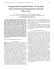### 35. APLIKASI LOGIKA FUZZY PADA OPTIMASI - Digilib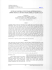### univerzita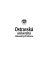### Epsilon Nets and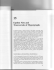### POSSIBILITY SINGLE VALUED NEUTROSOPHIC SOFT EXPERT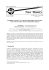### VENTILATORI ASSIALI